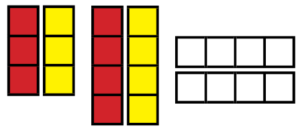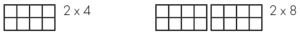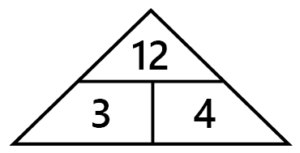# Getting started

Check the ACARA website for the most up-to-date Curriculum, as well as your state curriculum if needed.

Recent Changes

Version: 1.0 | Updated October 23 2019 | First release

## Basic Facts: Multiplication & Division Milestones Sheet

The Milestones provide a default position for schools to adopt a whole school approach for the development of properties and mental strategies associated with basic facts.

Even if you don’t adopt the Milestones steps, you can make use of the linked materials.

The Multiplication and Division Milestones have been designed such that the students learn the tables from easiest to hardest. Along the way on the easier tables they will pick up most of the harder tables “for free”, reducing the load later on.

# Year 2

#### Year 2 Prerequisites

Yr 2 ACMNA031: Recognise and represent multiplication as repeated addition, groups and arrays.

Yr 2 ACMNA032: Recognise and represent division as grouping into equal sets and solve simple problems using these representations.

Also Revisit:
– Groups
– Commutative Property of Multiplication
– Arrays

# Year 3

#### Year 3 Prerequisites

Yr 3 ACMNA056: Recall multiplication facts of two, three, five and ten and related division facts.

Books

Dice Dilemmas: Mary’s Game (pp. 20)

### Commutative Property of MultiplicationAny number multiplied by zero is zero. This can be illustrated through arrays. It is impossible to draw an array n x 0 or 0 x n.
This pattern needs to be made explicit.

Any number multiplied by one is itself (n x 1 = n).
This pattern needs to be made explicit.

Numbers may be multiplied in any order without affecting the product.

#### Relevant Materials

Books

Tackling Tables pp. 10,11

#### x2 Facts

Link the doubles addition facts learned in Year 2 to the x2 facts.
Introduce vocabulary such as double.

Teaching Tools:
Joining two sets of Unifix cubes of different colours will help make the links.Books

Tackling Tables pp. 39

#### x10 Facts

Pattern: End digit is zero. Double the fives facts.

#### Relevant Materials

Books

Tackling Tables pp. 24, 25

#### x5 Facts

Pattern: End digit is zero or five. Half the tens facts.

Books

Tackling Tables pp. 42

#### x3 Facts

Use known facts to derive new facts
e.g.
Use 6 x 2 = 12 to work out 6 x 3.
6 x 3 = (6 x 2) + 6.

Books

Tackling Tables pp. 40

# Year 4

#### Year 4 Prerequisites

Review facts learned in Year 3:
Multiplication Property of Zero (x0) facts,
Multiplication Property of One (x1) facts,
Commutative Property of Multiplication (2 x 3 = 3 x 2),
x2 facts,
x10 facts,
x5 facts
x3 facts

By the end of Yr 4: ACMNA075: Recall multiplication facts up to 10 × 10 and related division facts.
The grid shows the remaining facts to be learned.

#### x9 Facts

Show the pattern on a Number Grid.
Relate to 10 facts: e.g. 3 x 9 = (3 x 10) – 3

Books

Tackling Tables pp. 46

#### Doubling

x 4 facts are double x 2 facts, Similarly, x8 is Double x4

Books

Tackling Tables pp. 16

Dice Games for Tables (Entire book)

#### x4 Facts

Revise the 2x facts.
Link x 2 facts to x 4 facts.
(Doubling Strategy)

Books

Tackling Tables (pp. 41)

Dice Games for Tables (pp. 17-24)

#### x8 Facts

Link x 4 facts to x 8 facts. (Doubling Strategy)
or
Link x 2 facts to x 8 facts using double-double strategy.Books

Tackling Tables (pp. 45)

Dice Games for Tables (pp. 25-28)

#### x6 Facts

Link x 3 facts to x 6 facts.
(Doubling Strategy).

Books

Tackling Tables (pp. 43)

Dice Games for Tables (pp. 29-33)

#### x7 Facts

Last fact to learn (7 x 7).

This is a natural opportunity to point out the square numbers already learned (square numbers are covered in year 6).

Books

Tackling Tables (pp. 44)

#### Division Facts

Relate division to multiplication. e.g. show that: 4 x 3 = 12, 3 x 4 = 12
12 ÷ 3 = 4, 12 ÷ 4 = 3Books

Tackling Tables (pp. 44)

Check the Clues 2 ( )

Pocket Dice Book B (Diviso Game )

Pocket Dice Book C ( )

Dice Dazzlers ( )

Games

Mining Boom

Race Car Rally

Multispin (Multiplication 2-9)

Spindiv (Division 2-9)

Division Decision

#### Halving

Relate back to doubling

Divide by 4: halve and halve again (÷2, ÷ 2).
Divide by 8: halve, halve and halve again (÷ 2, ÷ 2, ÷ 2).

Books

Tackling Tables pp. 16

Dice Games for Tables (Entire book)

# Year 5

#### Continued Practise of Multiplication and Division Facts

Start of Year 5: An opportunity to review all basic multiplication and division facts to maintain recall

Yr 5 ACMNA098: Identify and describe factors and multiples of whole numbers and use them to solve problems.
Factors and Multiples.
Apply Simple Divisibility Tests.

Yr 5 ACMNA101: Solve problems involving division by a one digit number, …
Review all basic division facts.

Factors
For example,18 not just linked to one fact of 6×3 but has factors of 1, 2, 3, 6, 9 and 18.

Pocket Dice Book C

• Diviso Remainder pp.22-24

Games

Mining Boom

Race Car Rally

Multispin (Multiplication 2-9)

Spindiv (Division 2-9)

Division Decision

# Year 6

#### Fraction of a Quantity

Yr 6 ACMNA127: Find a simple fraction of a quantity where the result is a whole number …
Link to known multiplication and division facts.

3 x 4 = 12
4 x 3 = 12
12 ÷ 4 = 3
12 ÷ 3 = 4
1/3 of 12 = 4
1/4 of 12 = 3

Books

Pocket Dice Book C

• Fraction Of (pp.39-43)

Developing a Conceptual Understanding of Fractions

• pp. 62-72